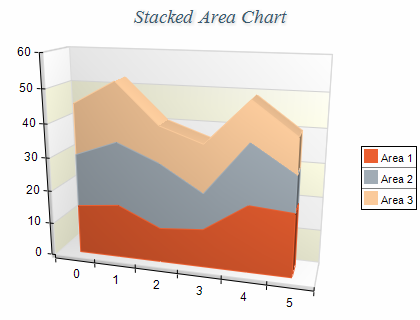﻿ Stacked Area
Nevron .NET Vision Documentation
Stacked Area

f

A Stacked Area chart displays related data groups, one on top of the other. It is used to show how each group contributes to the total as well as the trends of the total, usually over time. The series are displayed as sequences of straight line segments and the area below each line is filled. Each area series is stacked on top of the previous one. The height of the uppermost line depends on the total value for each category. The following figure displays a Stacked Area chart:Figure 1.

Creating a Stacked Area Chart

Stacked Area charts are displayed with several NAreaSeries objects. The MultiAreaMode property of the first area series must be set to MultiAreaMode.Series. The MultiAreaMode property of the subsequent area series must be set to MultiAreaMode.Stacked. The following example demonstrates how to create a stack area chart with two stacks:

C#
Copy Code
```// obtain a reference to the Cartesian chart that is created by default
NCartesianChart chart = (NCartesianChart)chartControl.Charts;

// create two area series

// stack the second area over the first one
area1.MultiAreaMode = MultiAreaMode.Series;
area2.MultiAreaMode = MultiAreaMode.Stacked;
```
Visual Basic
Copy Code
```' obtain a reference to the Cartesian chart that is created by default
Dim chart As NCartesianChart = chartControl.Charts(0)

' create two area series
Dim area1 As NAreaSeries = chart.Series.Add(SeriesType.Area)
Dim area2 As NAreaSeries = chart.Series.Add(SeriesType.Area)

' stack the second area over the first one
area1.MultiAreaMode = MultiAreaMode.Series
area2.MultiAreaMode = MultiAreaMode.Stacked
```

If you want to create a second stacked area with two stacks behind the stack area created in the previous sample you have to add the following code:

C#
Copy Code
```NAreaSeries area4 = (NAreaSeries)chart.Series.Add(SeriesType.Area);
area4.MultiAreaMode = MultiAreaMode.Series;
area5.MultiAreaMode = MultiAreaMode.Stacked;
```
Visual Basic
Copy Code
```Dim area4 As NAreaSeries = chart.Series.Add(SeriesType.Area)
Dim area5 As NAreaSeries = chart.Series.Add(SeriesType.Area)
area4.MultiAreaMode = MultiAreaMode.Series
area5.MultiAreaMode = MultiAreaMode.Stacked
```
Stack Origin
When an area series is stacked it is always displayed with origin 0, regardless of the values of the UseOrigin and Origin properties.
Stacks with negative values

By default stacked areas treat negative values with their absolute values. In order to use negative stack values you need to explicitly enable them by setting the AllowNegativeStackValues property to true:

C#
Copy Code
area.AllowNegativeStackValues = true;
Visual Basic
Copy Code
area.AllowNegativeStackValues = True
Formatting Commands

The following formatting commands inherited from the NSeries class have different meaning when an area series is stacked:

<total> - displays the absolute sum of the values in the current stack.
<cumulative> - displays the sum of the values up to the current stack value.
<percent> - displays the percent contribution of the value to the total pile sum.

Related Examples
Windows forms: Chart Gallery\Area\Stacked Area